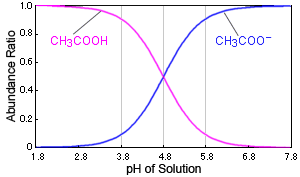# pKa and Dissociation Equilibrium

### 1. pH

When hydrochloric acid or other acid is added to water, the pH level decreases. The acidity of a solution is determined by its proton (hydrogen ion) concentration ([H+]), where pH provides a simple index for expressing the [H+] level. pH is indicated in terms of the following expression, where the smaller the number, the stronger the acidity (higher proton concentration).

One important point regarding pH is that a change of only 1 in the pH value is equivalent to a 10-fold change in proton concentration.

Note: Activity level should be used rather than concentration, but concentration was used instead of activity because concentration often corresponds to activity level in analytical concentrations and it is simpler (the same applies below).

### 2. pKa and Dissociation Equilibrium

Acids include strong acids, which completely dissociate in water, and weak acids, which only partially dissociate. When an acid dissociates, it releases a proton to make the solution acidic, but weak acids have both a dissociated state (A-) and undissociated state (AH) that coexist according to the following dissociation equilibrium equation.

The concentration ratio of both sides is constant given fixed analytical conditions and is referred to as the acid dissociation constant (Ka). Ka is defined by the following equation.

The square brackets indicate the concentration of respective components. Based on this equation, Ka expresses how easily the acid releases a proton (in other words, its strength as an acid). In addition, the equation shows how the dissociation state of weak acids vary according to the [H+] level in the solution.
Carboxylic acids (containing -COOH), such as acetic and lactic acids, normally have a Ka constant of about 10-3 to 10-6. Consequently, expressing acidity in terms of the Ka constant alone can be inconvenient and not very intuitive.
Therefore, pKa was introduced as an index to express the acidity of weak acids, where pKa is defined as follows.

For example, the Ka constant for acetic acid (CH3COOH) is 0.0000158 (= 10-4.8), but the pKa constant is 4.8, which is a simpler expression. In addition, the smaller the pKa value, the stronger the acid. For example, the pKa value of lactic acid is about 3.8, so that means lactic acid is a stronger acid than acetic acid.

### 3. pKa and Buffer Capacity

Another important point is the relationship between pH and the pKa of an acid. This relationship is described by the following equation.

This equation can be rearranged as follows.

This shows how pKa and pH are equal when exactly half of the acid has dissociated ([A-]/[AH] = 1). If the pH changes by 1 near the pKa value, the dissociation status of the acid changes by an extremely large amount.Fig. Relationship Between pH of Solution and Dissociation Status of Acetic Acid

In the case of acetic acid, for example, if the solution's pH changes near 4.8, it causes a large change in the presence of acetic acid. When the pH is 3.8, over 90 % exist as acetic acid molecules (CH3COOH), but at a pH of 5.8, over 90 % exist as acetate ions (CH3COO-).
Conversely, to change the pH level near the pKa value of an acid, the dissociation status of the acid must be changed significantly, which requires using an extremely large amount of acid or base. The ability of a substance to maintain the pH of such solutions is referred to as its buffer capacity, where the closer the pKa and pH are, the higher the buffer capacity. Therefore, when selecting a buffer solution, which are used widely in liquid chromatography, an acid or base buffer solution with a pKa value close to the target pH level is selected to maximize this buffer capacity.